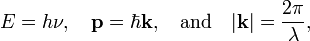# Photon

In physics, a photon is a massless elementary particle that travels with the speed of light and is associated with an electromagnetic wave.

Given an electromagnetic wave with wavelength λ, its frequency ν is inversely proportional to λ: ν = c/λ, where c is the speed of light (≈ 3·108 m/s). A photon is a light quantum with energy E and momentum p associated with this wave:$E = h \nu, \quad \mathbf{p} = \hbar \mathbf{k},\quad\mathrm{and}\quad |\mathbf{k}| = \frac{2\pi}{\lambda},$

where h is Planck's constant, ħ ≡ h/(2π) ≈ 1.055·10−34 Js, and k is the wave vector, a vector pointing in the direction of the propagation of the wave. Although a photon has linear momentum, it does not have rest mass.

The first to see that the electromagnetic field consists of energy parcels (light quanta) was Albert Einstein in 1905. Max Planck, five years earlier, assumed in his theory of blackbody radiation that a black body consists of material oscillators, and had made the revolutionary step that the energies of these oscillators are discrete, i.e., integral multiples of small energies—quanta. But Planck had not yet made the step to a quantized radiation field.

In 1923 Arthur Compton scattered X-rays off electrons and showed that the light quanta have—in addition to energy—linear momentum. Three years later, in 1926, Gilbert N. Lewis proposed the name photon for a "particle of light"—a light quantum. This was the same year that Erwin Schrödinger proposed his wave equation, which formed the basis of the new quantum mechanics. It took another year before Paul A.M. Dirac was able to fit the concept of the light quantum (photon) in the framework of the new theory.

In his 1927 paper Dirac quantized the electromagnetic radiation field, which means that he re-interpreted the classical electric and magnetic fields as quantum mechanical operators with well-defined commutation relations. The commutation relations of the field operators are those of bosons, particles of integer spin and he showed that photons have indeed integer spin S = 1. The spin multiplicity 2S+1 = 3 is given by two components corresponding to the two polarization directions of the electromagnetic wave and the third spin component corresponds to the propagation direction k.

See for more details: Quantization of the electromagnetic field.